﻿ houghton biology site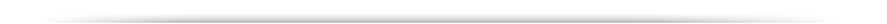Lecture 4: Genetic Linkage -Chromosome mapping -Tetrad analysis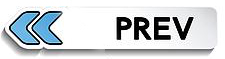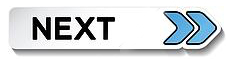Three point test crosses. Mathematically, two independent events happening at the same time-

However, to observe these traits three criteria had to be upheld...

1. Organism producing the crossover gametes must be heterozygous for all three traits.

2. The cross must be constructed so that each of the phenotypic traits must reflect a change in the genotype at each locus.

3. A sufficient number of offspring must be obtained to get statistically viable results.

In determining the gene order among three genes. You will need to know how this can be achieved!!

eg.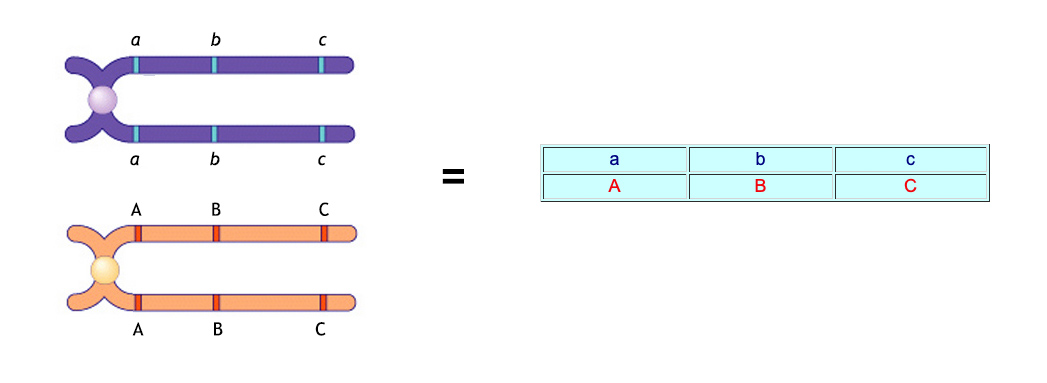F1:

 a b c A B C

This is equivalent to the required heterozygotic parental generation for all three traits.

Remember that this is the simplest case where all the wild-type alleles are dominant and, at least in this case, on the same chromosome.  Correspondingly, ALL the mutant alleles are iniitially on the other.

In the next generation these triple heterozygotes can be test- crossed with triply recessive testers (???)............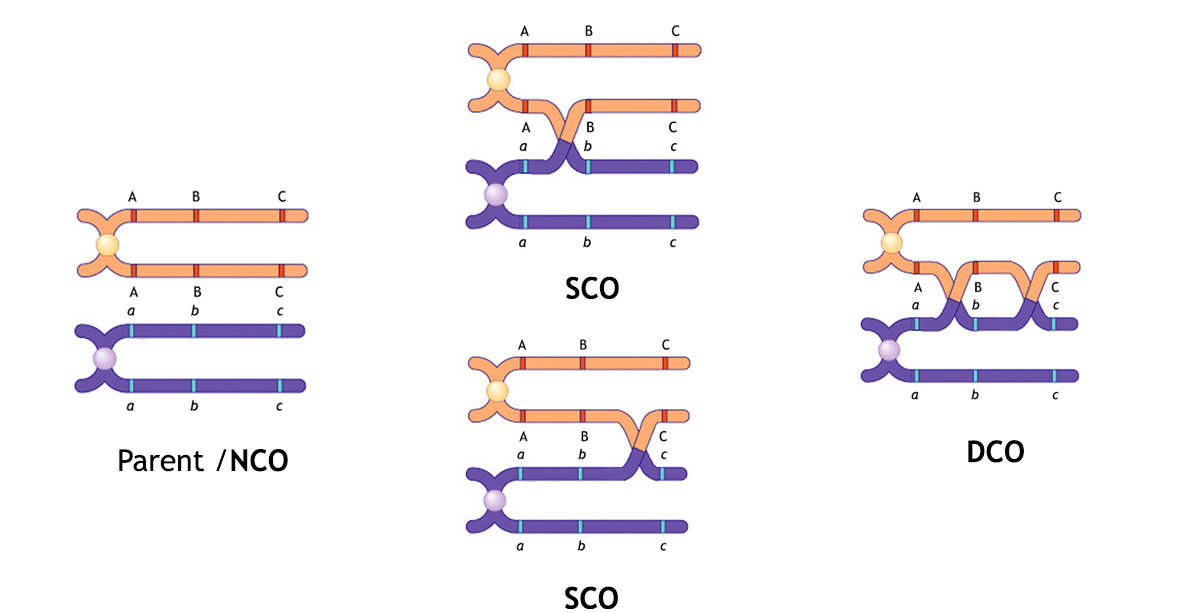In so doing, any "crossing-over" events that have occurred in the formation of the gametes will show through against the triply recessive pairing.

F2: listed as gametic genotypes...Crossover Types

 a b c = 230 = 467 = 42.1% = NCO A B C = 237 a B C = 82 = 161 = 14.5% = SCO A b c = 79 a b C = 200 = 395 = 35.6% = SCO A B c = 195 a B c = 44 = 86 = 7.8% = DCO A b C = 42

Thus, some vindication of the underlying linkage principle... but this all assumes the premise that the gene order is a b c

Another example: Cross of X/X y/y z/z with x/x Y/Y Z/Z is, however, not appear to behave is such an overtly simple manner, = -as there is now what is termed a mixed "association" of =Wild-Type and mutant alleles, giving:

F1

 X y z x Y Z

Now if this triply heterozygotic strain were to be three-point test crossed.. yielding 1448 total offspring

 X y z = 580 = 1172 = 80.9% = NCO x Y Z = 592 X y Z = 45 = 85 = 5.9% = SCO x Y z = 40 X Y z = 89 = 183 = 12.6% = SCO x y Z = 94 X Y Z = 3 = 8 = 0.5% = DCO x y z = 5

As we cannnot assume the alphabetical order of the three genes,..

the First question would be....... What is the gene order?  We find this out by looking at the NCO's and DCO's.

i.e. What would be the gene order that gives "X Y Z" as a DOUBLE crossover when the parentals associations are

"X y z" and "x Y Z"?

 X y z x Y Z

 y X z Y x Z

 X z y x Z Y

The answer is determined empirically by trial and error...   "y     x      z".

Now both the single cross overs (SCO) and double crossovers DCO numbers make sense.

 y X z = 580 = 1172 = 80.9% = NCO Y x Z = 592 y X Z = 45 = 85 = 5.9% = SCO Y x z = 40 Y X z = 89 = 183 = 12.6% = SCO y x Z = 94 Z X Y = 3 = 8 = 0.5% = DCO y x z = 5

The reorganization of the gene pairs further allows us to gauge the recombinant distance between each of the two sets of gene pairs giving:

Recombinant Frequency values (RF values) of 13.1%  for y and x, and 6.4% for x and z, respectively... remember you have to include the DCO values also!!.

The Second question: Are the relative rates of SCO's and DCO's consistent? If not... what is wrong?

Similarly, the Recombinant Frequency value (RF value) for recombination between the two outside genes pairs of y and z can also be determined by adding up the SCO values between y --- x and x --- z,

which equals 268 / 1448 =18.5%

Note, however, that the 13.1 + 6.4 = 19.5%, is a little higher than this calculated 18.5% -because the DCO's also needed to be considered when evaluating crossover frequency.

Morgan's student, Sturtevant, observed this phenomon in a number of their crosses, and rationally understood that the frequency of recombinants increased with increasing distance, and that it was more accurate to add the intervening distances from multiple small intervals, rather than rely on a single cross between two fairly distant (but linked) markers to determine the map distance.

This is not always the case, however.  The basic assumption being that the formation of a chiasma is a physical event and therefore as you analyze genes which are closer and closer together, there has to be a physical limit where steric hindrance in the formation of one synapse (at the synaptonemal complex) begins to interfere with the formation of the next.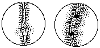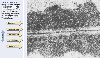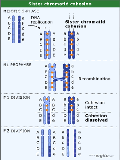To measure interference, we first calculate what is termed the coefficient of coincidence (c.o.c.), which essentially defines the ratio of observed to expected double recombinants.

Interference can then be calculated as 1 (unity) - c.o.c.

Along these same lines, assuming that there is enough room between the position of "linked" genes, if you can have one crossover between two loci residing on two non-sister chromatids then what's to stop there being more than one?

Again, as we have just discussed, if you have three loci that are close together, then you can perform the proverbial three point test cross, which ought to enhance your accuracy somewhat (and at the very least establish a gene order).

But what do you do if you don't have an appropriately defined third locus lying in between your two loci of choice? Moreover, what happens if you haven't got all the appropriate mutant strains.....

Given that it's all a matter of probabilities.... refer to statistics.

Use of a mathematical equation that was developed to predict the probability of random events... Poisson distribution.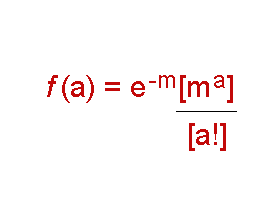where

a = the class of variables that you want to observe (the number of crossovers),

m = the mean number of items that you expect to get in your variations,

e = the base of the natural log

and ! = factorial function

Recombination frequency (RF) and the "ultimate mapping unit"....(m) can now be squeezed into this equation

In an assortment of genes where there is at least one crossover, we can contrast among all the different events.

The RF (frequency of recombinants) can be deduced empirically.

RF (if there are no crossovers) = 0

RF (if one crossover) recombinants ought to make up 50% of the possible products.

RF (if 2 crossovers) recombinants also appear to be pretty much the same....~ 50%.

Indeed, it turns out that it really doesn't matter how many recombinants you look for, the number of recombinants make up approximately one half of all the potential products of meiosis.

Therefore, the proportion of meioses with at least one crossover is

Unity (1) minus the fraction of those outcomes that have zero crossovers.

This "fraction" can be deduced through Poissonic logic by taking the value of "a" = 0,

giving the fraction = e-m [m0/0!] = e-m

Thus, RF (recombination frequency) = 0.5 x (1 -
e -m ).

which relates mapping units "m" (or the mean number of exchanges) directly to RF.

Now, if we express m as a "percentage fraction of crossovers" in order that we can equate it to centiMorgans (or the official map units) we must convert the value.....

Example: An empirically defined RF between two gene pair locations = 0.275 (27.5%).

plugging this value into the equation

=> 0.275 = 0.5 x (1 - e -m )

=> e-m =   1 - 0.55 = 0.45,

then to get a value for "m" go to a log table (which will always be provided in any test if necessary),

or available on-line ....

 Solve for "m" e-m = m = 0.45 0.8

But we are not quite finished yet.!!!!

We still need to convert this value to cM or real map units......

We have previously found that 100% (non-linkage) = 50 cM.

Thus, 50 cM is the highest number of centiMorgans you can get... effectively, therefore, all linked gene pairs at 50cM would behave according to Mendelian Principles, and not be subject to Poisson distributional analysis

Therefore, the true distance between two loci that DO experience linkage (in cM or map units)

=  50 x m cM.

.= 50 * 0.8 = 40cM, which (as it turns out) is considerably greater than the original value of 27.5 cM, that was empirically deduced

Use of mathematical equation that was developed to predict the "true" probability of random events... Poisson distribution.

RF (recombination frequency) = 0.5 x (1 - e -m ).

which relates mapping units "m" (or the mean number of exchanges) directly to RF.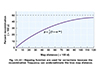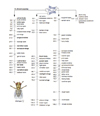Even so... recombinant maps rarely equate exactly to genetic maps.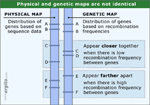It has always been curious to me that the discovery of "gene linkage" actually occurred using the sex chromomomes in Drosophila, which are the most dissimilar of all chromosomes and which highlight major unique features of sex-linkage...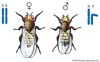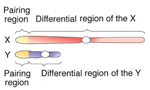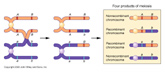What this means also, however, is that all the remaining chromosomes, the autosomal chromosomes, do recombine and form myriads of chiasmata each meiotic cycle (i.e. in germ-line cells in more complex eukaryotes, like ourselves). Consequently, it is inherently technically difficult to maintain "true" stocks of strains that are not being rearranged every time new gametes are being formed. Any potential control or forestalling of the inate chiasmata formation between homologous chromosomes that alway occurs in meiosis prophase I would be a huge advantage in maintaining stocks over several genarations. This potential is brought about the utilization/creation of what are called Balancer chromosomes, or rearranged chromosomes that can no longer line up appropriately in prophase 1 to allow for chiasmata to form.

These balancer chromosomes can be constructed synthetically and/or can occur naturally. Either way they can be used extensively to analyze the inheritance of genetic traits across generations without the interference of chiasmatic crossing over events exchanging information between similar reions of chromosomes.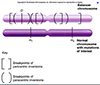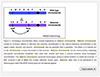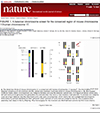There are other variables of gene "associations" that result from gene linkage and chromosomal misbehaviour.

Meiotic Nondysjunction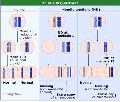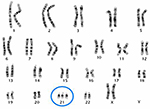Mitotic Nondysjunction.

(a) Calvin Bridges noticed that a strain of flies M M+ (heterozygous for "bristle" configuration), sometimes expressed itself as a "local variant" of the dominant M allele (slender bristle) in which a region of the fly’s body exhibited wild-type (M+) bristle characteristics.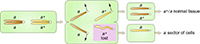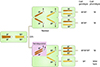He attributed this phenomenon to a genetic event termed mitotic nondysjunction, where one half of the resulting progeny of mitosis have either three copies or only one copy of the chromosome (resulting from an aberrant mitotic division).

Sometimes such a localized phenotypic variation can occur as a result of chromosome loss during mitosis of a somatic or non-germ-line cell.

Either way, the local variation was, and is, thought to result from a catastrophic loss or abnormal migration of whole chromosomes.

Segregation at the phenotypic level, i.e. heterozygotes exhibiting localized "patches" of variant phenotypes.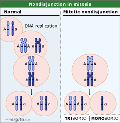(b) In 1936 Curt Sterns (another student of Morgan's working on D. melanogaster) undertook a cross of two other sex-linked traits y (yellow hair) and sg (singed hair).

y+ sn / y+ sn (singe-haired female) with y sn+ / Y (yellow singe-haired male).

Not too surprisingly the female progeny were mostly Wild-Type with grey bodies and normal bristles y+ sn / y sn+

Occasionally, however, Stern noticed that there were some localized "twin spots" of apparently "coupled" yellow and singed hair (y sn) phenotypes, which occurred as thes associative spots too often as to be a consequence of mere coincidental juxtapositioning. How might these be explained?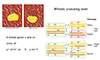He accounted for this by asserting the phenomenon of mitotic recombination, i.e. the chance occurrence of a crossing over event occurring between two regions of a homologous chromosomes during MITOSIS (see above). These regions, he reasoned, do not have to be BETWEEN the gene loci. Indeed, in this instance Sterns hypothesized that the crossing over event occurs between one of the gene loci and the centromere.

N.B. Don't forget that diploid cells give rise to diploid progeny in mitotic divisions!!

Sterns' work was the first example of "genetic mosaicism", which is the presence of two or more populations of cells within the same organism with different genotypes. It was the birth of what is now a significnt branch of molecular genetics called "mosaic analysis", which is one of the major tools used today to analyze the effects of lethal or "specifying" genes in the development of a number of organisms, by allowing geneticists to compare the genetic heritage of two cell lines -with the only difference between the two being a somatic aberration -that can often be induced.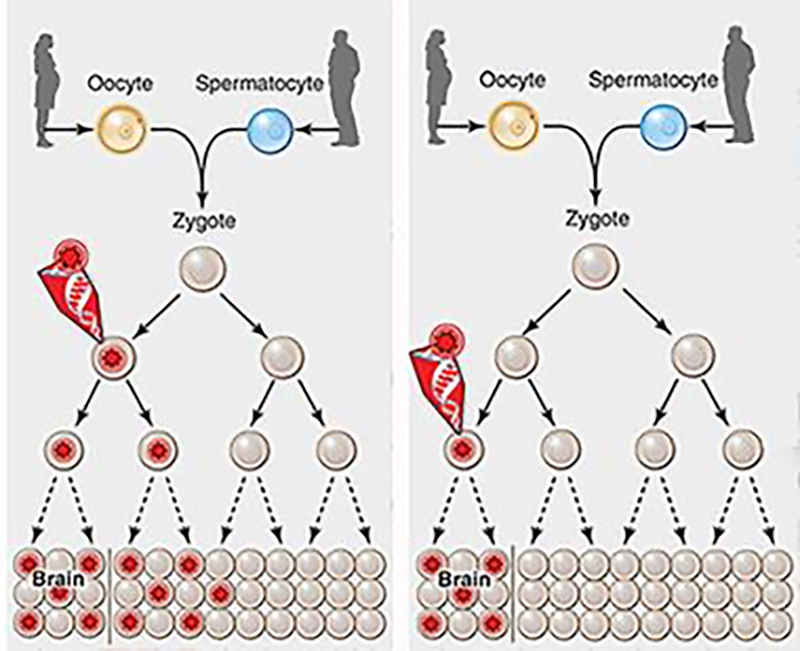Additional concerns with genetic exchange through crossing over.Consider a pair of homologous chromosomes prior to the MI division which contain a single crossover in the interval between markers B and D on chromatids 2 and 3, as shown in the Figure below. The outcome of the single crossover is two chromatids carrying a recombination of the phenotypic markers associated with the B and D genes. Now, consider that a second crossover occurs in this same interval. There are four ways this could occur, and each of these is equally probable.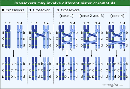• (Case 1):   As we have alrady dealt with.  The second crossover could occur again between chromatids 2 and 3, restoring the original configuration of markers B and D. If the investigator were only analyzing recombination of B and D, then the double crossover involving two strands would appear as though there had been no crossover at all: it would be indistinguishable from the 0 crossover class. Only if the investigator follows marker C in this example would the double crossover be apparent, providing a reciprocal recombination event of B-c-D and b-C-d.

• (Cases 2 and 3): The second crossover could involve only one of the original chromatids. The first crossover involved chromatids 2 and 3, so the second crossover could involve 1 and 3, or 2 and 4. (Recall that the meiotic crossover predominantly occurs between homologous chromosomes, and not between sister chromatids. Therefore, it is unlikely to occur between 1 and 2, or between 3 and 4.) This double crossover, involving three strands, gives the same recombination of the phenotypic markers as in the single crossover (only two chromatids show a recombination between B and D) and can only be distinguished from the single crossover class by analysis of the C marker.

• (Case 4): Finally, the second crossover could occur between chromatids 1 and 4, neither of which were involved in the first crossover. This case is unique, since it results in four recombinant chromosomes and no wild type associations. This feature is particularly powerful in identifying the frequency of double crossovers: this class can often be identified unambiguously, and represents 1/4 * the total number of double crossovers. Using tetrad analysis to determine gene-gene linkage for an example).

How do we analyze for this and assure ourselves that each non-sister chromatid has an equal chance of crossing over with each of the other paired non-sister chromatids.

Yeast and fungi.

S. cerevisiae: and the potential POWER of yeast genetics -provides relatively immediate proof of all types of chiasmata and their consequences. Use of yeast and other similar fungi have many distinct advantages ...S. cerevisiae can grow mitotically in a stable fashion as either a haploid (with one copy of each chromosome) or a diploid (with two copies of each chromosome).  In essence, therefore, the consequences of meiosis can be "harvested" through analysis of both states, and the direct products of meoiotic events can be analyzed.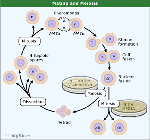Genetic Orderliness in Ordered and Unordered Tetrad analyses

The power of tetrad analysis comes from the fact that all four meiotic products are contained within a single ascus.

The most fundamental test that can be quickly achieved using tetrad analysis is the one for single-gene segregation.

This can determine whether a mutant phenotype is caused by a mutation in a single gene or not. If a single gene is the cause, the mutation should segregate within the tetrads in a predictable, Mendelian fashion for a single gene.

All fungi and algae form "haploid" spores, that contain 4 or 8 spores, resulting from a SINGLE meiotic division.

These result in "ordered" or "unordered" arrangement of spores within their respective asci.

As we will see later, linear tetrad analyses can also allow for "centromere mapping" in "ordered" asci, or a determination of the distance from any given gene locus to its centromere (again using recombination as a tool to determine distance).

In choosing two linked genotypes that are easily discernible (phenotypes) this can give rise to a simple analysis of meiotic function.

e.g.                   a       b          x          a+        b+

Three different assortment options no matter whether the two genes are linked or not

 1 2 3 a b a b+ a b a b a b+ a b+ a+ b+ a+ b a+ b a+ b+ a+ b a+ b+

Parental Ditype (PD).......Non-Parental Ditype(NPD) ..........Tetra-Type (TT)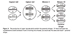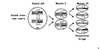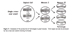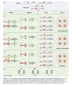• A parental ditype (PD) is a tetrad in which two genetic markers are segregating, and two spores have one parental genotype and two spores have the other parental genotype.

• A nonparental ditype (NPD) is a tetrad in which two genetic markers are segregating, and two spores have one nonparental genotype and two spores have the other nonparental genotype.

It turns out that testing the possible linkage of two gene mutations with unknown map positions is relatively simple.., the fundamental question to answer is,

"Does the number of PD's equal the number of NPD's?"

If the answer is YES, the association of mutations (and hence the gene loci) are unlinked.

If the answer is NO, they are probably linked.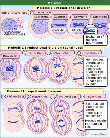Meiosis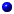The first class of tetrad is that in which the two parental sets of markers segregate away from each other at meiosis I.

The resulting tetrads have two spores bearing the genotype of one parent (HIS4 - trp1) and the other two spores bearing the genotype of the other parent.

This class of tetrad, having the two classes of parental spores, is called a parental ditype (PD),

and this arrangement of spores in the ascus can be acheived if genes are on different chromosomes or on the same chromosome -as per Mendel.

But let's go through this tetrad analysis with two genes on different chromosomes and "linked" genes

The second class of tetrad is that in which segregation at meiosis I occurred in the opposite fashion. The result is a tetrad with two different classes of spores. This class of tetrad, having two classes of nonparental spores, is called a nonparental ditype (NPD)

Similarly, this arrangement of spores in the ascus can be acheived if genes are on different chromosomes or on the same chromosome.

Because segregation at meiosis I is "random" and occurs at equal frequency (in accordance with Mendel's First Law), for two unlinked genes, the frequency of PD tetrads should equal the frequency of NPD tetrads

(PD = NPD).

This prediction constitutes one of the fundamental tests of genetic linkage in yeast.

The third type of tetrad arises as the result of a crossing over, or recombination, between one of the genes and its centromere. Crossing over during yeast meiosis occurs after replication, when there are four chromatids.

For two unlinked genes on different chromosomes the frequency of TT tetrads will depend on the linkage of at least one of the gene to its centromere, as it can ONLY result from a crossing over of either gene with respect to its centromere. This property can ultimately be used to determine whether a gene is linked to its centromere or not.

For two unlinked genes on the same chromosome a TT tetrad can arise as the result of a crossover between one of the gene markers and its centromere, and is even less likely and occurrence.

---

Now let us consider a different type of tetrad analysis, in which two genes are now "LINKED" to each other on the same chromosome.

By tetrad analysis we can determine the degree of genetic linkage between such markers.

In the extreme cases of two genes that are completely linked, with no detectable recombination between them, we expect that tetrad analysis will reveal that ALL the tetrads are PD tetrads.

What about cases where the two genes are linked but there is some recombination between them?

In those cases, the number of PD tetrads will be greater than the number of NPD tetrads (PD > NPD). This is because PD tetrads ONLY arise from no recombination.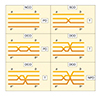In contrast, for two linked genes, NPD tetrads can also arise by a double crossover between the two linked markers in a three stranded exchange). As for centromere linkage, in the case of closely linked genes, we can calculate the degree of linkage based on the frequencies of the different types of tetrads produced.
..

A single crossover between linked markers produces a TT tetrad.

Recombination (SCO's and DCO's) between linked genes can also produce PD, NPD, and TT tetrads.

-Recall that for two unlinked genes, when PD = NPD, TT tetrads can also arise by a crossover between a gene and its centromere.

However, in the case of linked genes, when PD > NPD, TT tetrads can arise by a single crossover between the two genes and also by two of the four types of double crossovers, as shown in the multiple crossover figure.It is, therefore, possible to relate T(etraTypes) and NPD' s (non-parental ditypes) to the potential single crossover events (SCO's) and double crossover events (DCO's).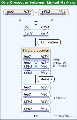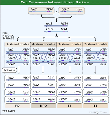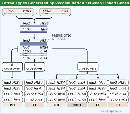With "linked" genes, however, there is only ONE way to obtain an NPD -through a double cross over.

Thus, with respect to the NPD’s .......... the number of DCO's obtained = 4NPD

In a similar way, SCO can be written as being = T - 2NPD, as there are three ways to get a TT (Tetratype; 2 of which are DCO's).

Now, from a previous understanding of crossover frequencies

NCO = 1(or unity) - (SCO + DCO)

(assume that triple, quadruple crosses etc can generally be ignored as having less than a significant affect upon the end results)

let m = the mean no. of crossovers, thus m = SCO + 2 x DCO (as the actual number of crossovers in a double cross is 2).

substitute appropriate values:

=> m = (T-2NPD) + 2(4NPD) = T + 6NPD

then (as always) one needs to convert to cM by multiplying by 50 =>

now RF (in cM) = 50 (T + 6NPD)

Chromatid interference.

Note: The theoretically maximal value for RF being equal to 50 can only be mathematically true if and when the potential for chromatid interference is not a "significant" factor in the calculation, i.e. the gene pairs are sufficiently far enough apart that the effect of chromosome interference is negligible.Consequently, if you use the formula RF = 50 (T+6NPD) (from the above definition) then the occurrence of chromatid interference might conceivably overestimate the RF value, which could therefore (in practice) numerically exceed 50%!!!!!

___________________

Centromere mapping. While unordered tetrads allow for a rapid and relatively simple evaluation of recombination frequencies (and therefore genetic linkage of proximal genes present on the same chromosome, analysis of ordered tetrads (or octads) allows for an additional evaluation of the proximity of genes to the centromere.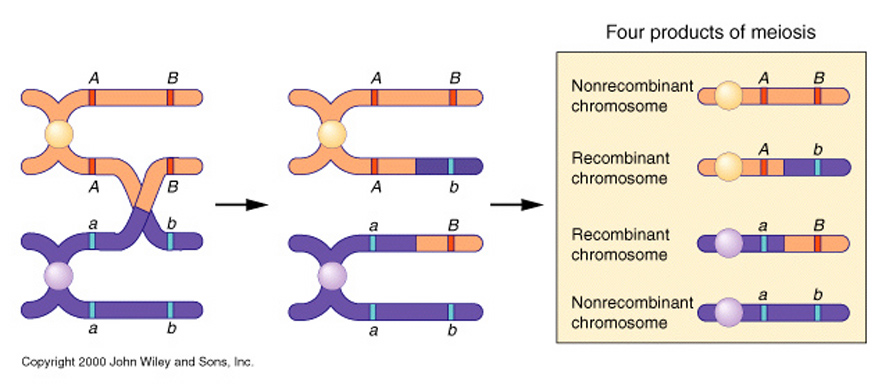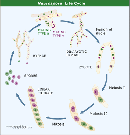Neurospera crassa or Sordaria sp. are examples of fungi that gives rise to an ordered arrangement of its spores within their respective ascospore. Specific variations in the location of given trait within the ascospore leaves a tell-tale assortment of an allele that is due to a crossing over event between the centromere and a given gene locus. In a similar manner to regular crossing over phenomena the distance between the centromere and a gene can thus be estimated by analyzing the frequency of "second division" segregation within the ordered tetrad (octad) and dividing by 2 (as only half of the resulting allele locations are due to this crossing over event).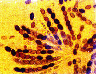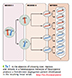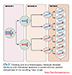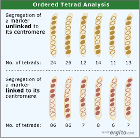e.g. in the case of a crossover between the A locus and the centromere.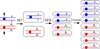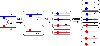A a A a A a A a A a A a A a a A a A A a a A a A a A A a a A a A A a a A a A a A A a a A a A A a _____ _____ _____ _____ _____ _____ 126 132 9 11 10 12

Total = 300

The recombinant forms are: 9 + 11 + 10 + 12 = 42  =  (42 / 300) x 100 = 14% are recombinants.

Now divide by two to get cM

=> Locus A therefore lies 7cM from the centromere.

Complications........ it’s always the exceptions that "proves" the rule!

-------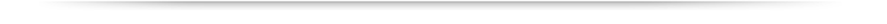Copyright © Department of Biology, Georgia State UniversityView Legal Statement Contact Biology Office: Tel: 404-413-5300
 About Academics Admissions Research Libraries About University Library Campus Life Athletics Alumni